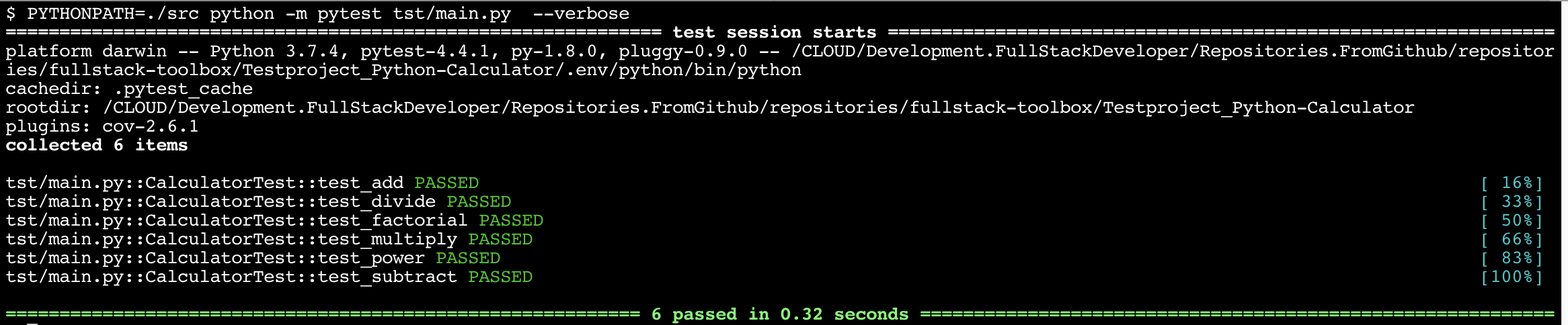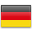# Python | Test-Driven Development

• Part 1: Create a TDD Python Project
• Part 2: Use Jenkins to automatically test your App

## Part 1: Create a TDD Python Project

Final source code is on Github.

## Introduction

The task of creating an error free program is not easy. And, if your program runs free of errors, keeping it error-free after an update or change is even more complicated. You don’t want to insert new errors or change correct code with wrong parts.

The answer to this situation (directly from the Oracle of Delphi) is: Testing, Testing, Testing

This means: think about what the result should be and then create a Test that checks this. Imagine, you have to write a function for adding two values, and you should describe the functionality.

So, maybe, your description contains one or two examples:

My functions add’s two numbers, e.g 5 plus 7 is 12 (or at least should be 12 :))

The procedure with the TDD is:

• think and define, what the function should to
• write a stub for the function, e.g. only function parameters and return type
• write a function, that tests you function with defines parameters and know result

For our example above, this means:

Write the python script with the desired functionality: `src/main.py`

```def add(val1,val2):
return 0 # this is only a dummy return value```

Write the Python Testscript: `tst/main.p`

```def_test_add():

if (result = 12):
print("everything fine")
else:
printf("ups, problems with base arithmetics")```

Now, with these in your toolbox, you can always verify your code by running the tests.

```\$ python test_add.py
ups, problems with base arithmetics```

dfdf

## Setup virtual environment

Mostly, tests are repeated after every change. So, to be sure, that each test is running the same way and with the same environment, we will use pythons virtual environment feature to create a new fresh python environment for the tests.

### Create virtual environment

`\$ python3 -m venv .env/python`

### Activate environment

Add the following line to .bashrc (or .envrc if you are using direnv)

`\$ . .env/python/bin/activate`

Install required packages

`\$ pip install pytest`

## Create a sample Application

### Prepare folder

Create folder for sources

`\$ mkdir src`

Create sample package

```\$ mkdir src/CalculatorLib
\$ touch src/CalculatorLib/__init__.py
\$ touch src/CalculatorLib/Calculator.py```

At least, create a simple Calculator: `src/CalculatorLib/Calculator.py`

```class Calculator:
def __init__(self):
print("Init Calculator")

return a + b

def subtract(self, a, b):
return a - b

def multiply(self, a, b):
return a * b

def divide(self, a, b):
return a / b

def power(self, base, exp):
return base ** exp```

Create the Main App for your Calculator: `src/main.py`

```from CalculatorLib.Calculator import Calculator

class Main(object):

def run(self):
c = Calculator()

print("5 + 3 = %5d" % c.add(5, 3))
print("8 - 4 = %5d" % c.subtract(8, 4))
print("5 * 3 = %5d" % c.multiply(5, 3))
print("8 / 4 = %5d" % c.divide(8, 4))

print("8 ^ 4 = %5d" % c.power(8, 4))

if __name__ == '__main__':
Main().run()```

Yur done with the fist development step. Try your app:

```\$ python src/main.py
Init Calculator
5 + 3 =     8
8 - 4 =     4
5 * 3 =    15
8 / 4 =     2
8 ^ 4 =  4096```

We will start with our first test. Create folder for tests and a file `tst/main.py`

```\$ mkdir tst
\$ touch tst/main.py```

Use the following for your test script `tst/main.py`

```from CalculatorLib.Calculator import Calculator
import unittest

class CalculatorTest(unittest.TestCase):

@classmethod
def setUpClass(self):
self.c = Calculator()

def test_subtract(self):
self.assertEqual(4, self.c.subtract(8, 4))

def test_multiply(self):
self.assertEqual(32, self.c.multiply(8, 4))

def test_divide(self):
self.assertEqual(2, self.c.divide(8, 4))

def test_power(self):
self.assertEqual(16, self.c.power(2, 4))

if __name__ == '__main__':
unittest.main()```

```\$ PYTHONPATH=./src python -m pytest tst/main.py  --verbose
================================= test session starts ================================
platform darwin -- Python 3.7.4, pytest-4.4.1, py-1.8.0, pluggy-0.9.0 -- <Testproject_Python-Calculator/.env/python/bin/python>
cachedir: .pytest_cache
rootdir: <Testproject_Python-Calculator>
plugins: cov-2.6.1
collected 5 items

tst/main.py::CalculatorTest::test_divide PASSED          [ 40%]
tst/main.py::CalculatorTest::test_multiply PASSED        [ 60%]
tst/main.py::CalculatorTest::test_power PASSED           [ 80%]
tst/main.py::CalculatorTest::test_subtract PASSED        [100%]```

The command to run the test is `python -m pytest tst/main.py,` but why the lead Variable `PYTHONPATH`?

Try it without:

```\$ python -m pytest tst/main.py
=================================== test session starts ==================================
platform darwin -- Python 3.7.4, pytest-4.4.1, py-1.8.0, pluggy-0.9.0 -- ##/Testproject_Python-Calculator/.env/python/bin/python
cachedir: .pytest_cache
rootdir: ##/Testproject_Python-Calculator
plugins: cov-2.6.1
collected 0 items / 1 errors

========================================= ERRORS =========================================
____________________________________ ERROR collecting tst/main.py ________________________
ImportError while importing test module '##/Testproject_Python-Calculator/tst/main.py'.
Hint: make sure your test modules/packages have valid Python names.
Traceback:
tst/main.py:2: in <module>
from CalculatorLib.Calculator import Calculator
E   ModuleNotFoundError: No module named 'CalculatorLib'
!!!!!!!!!!!!!!!!!!!!!!!!! Interrupted: 1 errors during collection !!!!!!!!!!!!!!!!!!!!!!!!
================================== 1 error in 1.84 secon==================================
```

Recognize the ModuleNotFoundError in line 16! This means, that Python could not find the desired CalculatorLib.

```\$ tree .
.
├── src
│   ├── CalculatorLib
│   │   ├── Calculator.py
│   │   ├── init__.py
│   └── main.py
└── tst
└── main.py```

.

In your Testscript, we import the CalculatorLib whit this statement:

`from CalculatorLib.Calculator import Calculator`

Python is interpreting this in the following way:

• Look in the folder of the test script for a subfolder with the name CalculatorLib
• There, look for a file `Calculator.py`
• And in this file, use the class Calculator

Obviously, the folder `CalculatorLib` is NOT in the same folder as the test script: it is part of the `src` folder.

So, using the environment variable `PYTHONPATH`, we inform python where to search python scripts and folders.

Add a function at the end of your Calculator: `src/CalculatorLib/Calculator.py`

```    ....
def factorial(self, n):
return 0```

Add a call of the new function to your main app: `src/main.py`

```    ...
def run(self):
...
print("4!    = %5d" % c.factorial(4))```

Add a test for the new function to your test script: `tst/main.py`

```    ...
def test_factorial(self):
self.assertEqual(24, self.c.factorial(4))```

Try it:

```\$ python src/main.py
Init Calculator
5 + 3 =     8
8 - 4 =     4
5 * 3 =    15
8 / 4 =     2
8 ^ 4 =  4096```
```\$ PYTHONPATH=./src python -m pytest tst/main.py
==================================== test session starts =====================================
platform darwin -- Python 3.7.4, pytest-4.4.1, py-1.8.0, pluggy-0.9.0
rootdir: ##/Testproject_Python-Calculator
plugins: cov-2.6.1
collected 6 items

tst/main.py ..F...                                                                      [100%]

========================================== FAILURES ==========================================
_______________________________ CalculatorTest.test_factorial ________________________________

self = <main.CalculatorTest testMethod=test_factorial>

def test_factorial(self):
>       self.assertEqual(24, self.c.factorial(4))
E       AssertionError: 24 != 0

tst/main.py:31: AssertionError
============================= 1 failed, 5 passed in 0.14 seconds =============================```

Test failed, was we expect it.

Now, implement the function correctly and startover the test:

Add a function at the end of your Calculator: `src/CalculatorLib/Calculator.py`

```import math

class Calculator:
...
def factorial(self, n):
if not n >= 0:
raise ValueError("n must be >= 0")

if math.floor(n) != n:
raise ValueError("n must be exact integer")

if n+1 == n:  # catch a value like 1e300
raise OverflowError("n too large")

result, factor = 1, 2

while factor <= n:
result *= factor
factor += 1

return result```
```\$ PYTHONPATH=./src python -m pytest tst/main.py  --verbose
==================================== test session starts =====================================
platform darwin -- Python 3.7.4, pytest-4.4.1, py-1.8.0, pluggy-0.9.0 -- ##/Testproject_Python-Calculator/.env/python/bin/python
cachedir: .pytest_cache
rootdir: ##/Testproject_Python-Calculator
plugins: cov-2.6.1
collected 6 items

tst/main.py::CalculatorTest::test_divide PASSED                                          [ 33%]
tst/main.py::CalculatorTest::test_factorial PASSED                                       [ 50%]
tst/main.py::CalculatorTest::test_multiply PASSED                                        [ 66%]
tst/main.py::CalculatorTest::test_power PASSED                                           [ 83%]
tst/main.py::CalculatorTest::test_subtract PASSED                                        [100%]

================================== 6 passed in 0.01 seconds ==================================```

## Testing Frameworks

#### Unit testing framework

```import unittest

class TestStringMethods(unittest.TestCase):

def test_upper(self):
self.assertEqual('foo'.upper(), 'FOO')

def test_isupper(self):
self.assertTrue('FOO'.isupper())
self.assertFalse('Foo'.isupper())

def test_split(self):
s = 'hello world'
self.assertEqual(s.split(), ['hello', 'world'])

with self.assertRaises(TypeError):
s.split(2)

if __name__ == '__main__':
unittest.main()```

#### pytest – helps you write better programms

```# content of test_sample.py
def inc(x):
return x + 1

assert inc(3) == 5```
`\$ pytest`

#### nose – is nicer testing for python

```def test_numbers_3_4():
assert multiply(3,4) == 12

def test_strings_a_3():
assert multiply('a',3) == 'aaa```

#### Python BDD Pattern

```class MangoUseCase(TestCase):
def setUp(self):
self.user = 'placeholder'

@mango.given('I am logged-in')
def test_profile(self):
self.given.profile = 'profile'
self.given.photo = 'photo'

@mango.when('I click profile')
def when_click_profile():
print('click')

@mango.then('I see profile')
def then_profile():
self.assertEqual(self.given.profile, 'profile')

@mango.then('I see my photo')
def then_photo():
self.assertEqual(self.given.photo, 'photo')```

#### radsh is not just another BDD tool …THE ROOT FROM RED TO GREEN

```from radish import given, when, then

@given("I have the numbers {number1:g} and {number2:g}")
def have_numbers(step, number1, number2):
step.context.number1 = number1
step.context.number2 = number2

@when("I sum them")
def sum_numbers(step):
step.context.result = step.context.number1 + \
step.context.number2

@then("I expect the result to be {result:g}")
def expect_result(step, result):
assert step.context.result == result```

#### doctest

```"""
The example module supplies one function, factorial().  For example,

>>> factorial(5)
120
"""

def factorial(n):
"""Return the factorial of n, an exact integer >= 0.

>>> [factorial(n) for n in range(6)]
[1, 1, 2, 6, 24, 120]
>>> factorial(30)
265252859812191058636308480000000
>>> factorial(-1)
Traceback (most recent call last):
...
ValueError: n must be >= 0

Factorials of floats are OK, but the float must be an exact integer:
>>> factorial(30.1)
Traceback (most recent call last):
...
ValueError: n must be exact integer
>>> factorial(30.0)
265252859812191058636308480000000

It must also not be ridiculously large:
>>> factorial(1e100)
Traceback (most recent call last):
...
OverflowError: n too large
"""

import math
if not n >= 0:
raise ValueError("n must be >= 0")
if math.floor(n) != n:
raise ValueError("n must be exact integer")
if n+1 == n:  # catch a value like 1e300
raise OverflowError("n too large")
result = 1
factor = 2
while factor <= n:
result *= factor
factor += 1
return result

if __name__ == "__main__":
import doctest
doctest.testmod()```

## Sample Session with Test Frameworks

```\$ py.test -v
========================================================= test session starts ==========================================================
platform darwin -- Python 3.7.3, pytest-4.3.1, py-1.8.0, pluggy-0.9.0 -- /CLOUD/Development.Anaconda/anaconda3/bin/python
cachedir: .pytest_cache
rootdir: /CLOUD/Development.Python/Repositories.FromGithub/repositories/python-toolbox/Working-with-TDD/app, inifile:
plugins: remotedata-0.3.1, openfiles-0.3.2, doctestplus-0.3.0, arraydiff-0.3
collected 4 items

test_base.py::test_should_pass PASSED                                                                                            [ 25%]
test_base.py::test_should_raise_error PASSED                                                                                     [ 50%]
test_base.py::test_check_if_true_is_true PASSED                                                                                  [ 75%]
test_base.py::test_check_if_inc_works PASSED```
```\$ nosetests -v
test_base.test_should_pass ... ok
test_base.test_should_raise_error ... ok
test_base.test_check_if_true_is_true ... ok
test_base.test_check_if_inc_works ... ok

----------------------------------------------------------------------
Ran 4 tests in 0.001s

OK```German